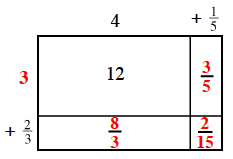### Home > CC1MN > Chapter 5 Unit 5B > Lesson CC1: 5.1.4 > Problem5-34

5-34.

Complete the diagram below and write the multiplication problem and answer that would go with it. Homework Help ✎

 $4$ $+\frac{1}{5}$ $12$ $+\frac{2}{3}$

Divide 12 by 4 to find the missing dimension of the rectangle.

$\frac{12}{4}=3$

Then find the other dimensions using multiplication.

$12+\frac{3}{5}+\frac{8}{3}+\frac{2}{15}$